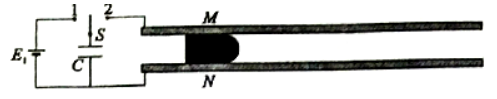$\text{A.}$ 匀强磁场的方向垂直导轨平面向下 $\text{B.}$ $M N$ 刚开始运动时的加速度 $\frac{B\left(E_1+E_2\right) L}{m R}$ $\text{C.}$ $M N$ 离开导轨后的最大速度为 $\frac{B\left(E_1-E_2\right) L C}{m}$ $\text{D.}$ $M N$ 离开导轨后的最大速度为 $\frac{B\left(E_1+E_2\right) L C}{m}$